# Timeage

Seven times of my age is 8 less than the largest two-digit number. How old I am?

Correct result:

x =  13

#### Solution:

7x = 99-8

7•x = 99-8

7x = 91

x = 13

Our simple equation calculator calculates it.We would be pleased if you find an error in the word problem, spelling mistakes, or inaccuracies and send it to us. Thank you!Tips to related online calculators
Do you have a linear equation or system of equations and looking for its solution? Or do you have quadratic equation?
Do you want to convert time units like minutes to seconds?

## Next similar math problems:

• Ravens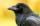The tale of the Seven Ravens were seven brothers, each of whom was born exactly 2.5 years after the previous one. When the eldest of the brothers was 2-times older than the youngest, mother all curse. How old was seven ravens brothers when their mother cu
• Father and son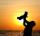Father is three times older than his son. 12 years ago father was nine times older than the son. How old are father and son?
• Mother and daughter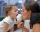Three years ago mother was three times older than daughter. After nine years she will be only twice old. How old is mother (and daughter)?
• Three siblings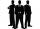Three siblings have birthday in one day-today. Together they have 35 years today. The youngest is three years younger than middle and the oldest is 5 years older than middle. How old is each?
• Three friends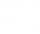Peter is eight times older than Rado, Rado is 3 times younger than Joseph. Together they have 120 years. Determine how many are each of them.
• Age questionJano is three times older than Zdeno. Zdeno is 12 years younger than Janko. How old is Jano and how old is Zdeno?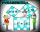Two fifth-graders teams competing in math competitions - in Mathematical Olympiad and Pytagoriade. Of the 33 students competed in at least one of the contest 22 students. Students who competed only in Pytagoriade was twice more than those who just compete
• Scouts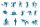Three scouts went on a three day trip. The second day went 4 km more than the first day. The third day went two times less than the first day. They went along 54 km. How many kilometers went every day together?
• Turtles Žofka and Julka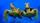Gray wolf kept in his tent turtles Žofka and Julka, which together have 200 years. After 50 years will Julka 2 times older than Žofka. How old are the turtles?
• Two math problems1) The sum of twice a number and -6 is nine more than the opposite of that number. Find the number. 2) A collection of 27 coins, all nickels, and dimes, is worth \$2.10. How many of each coin are there? The dime, in United States usage, is a ten-cent coin.
• Motion2Cyclist started out of town at 19 km/h. After 0.7 hours car started behind him in the same direction and caught up with him for 23 minutes. How fast and how long went car from the city to caught cyclist?
• Two-digit numberDigit sum of thinking two-digit natural number is 11. When it exchanging a sequence of digits, given a number which is 27 less than the thinking number. Find out which number I think.
• Two friends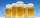Two friends met as a good man perish together for a beer. After recovery the most important topics (politics, women, football ...), one asks: - And how many do you have children? - I have 3 children. - And how many years have? Friend already not want to a
• Time goneSquare of Richard age equals the age of his mother. When he will bw two times older then his mom will be 7/2 times older than he. How old is Richard and his mom?
• Age problemsA) Alex is 3 times as old as he was 2 years ago. How old is he now? b) Casey was twice as old as his sister 3 years ago. Now he is 5 years older than his sister. How old is Casey? c) Jessica is 4 years younger than Jennifer now. In 10 years, Jessica will
• ExcavationMr. Billy calculated that excavation for a water connection dig for 12 days. His friend would take 10 days. Billy worked 3 days alone. Then his friend came to help and started on the other end. On what day since the beginning of excavation they met?
• Scouts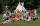The boys from scout group traveled 5 days distance 115 km. Every day walked 1.5 km less than the previous day. How many kilometers scouts walked in the first day?